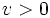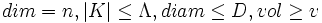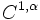# Convergence theorem

## Statement

Let$n \in \mathbb{N}$,$\Lambda < \infty$,$D < \infty$ and$v > 0$.

Let$M_j$ be a sequence of Riemannian manifolds such that each$M_j$ satisfies:$dim = n, |K| \le \Lambda, diam \le D, vol \ge v$

The$M_j$ have a subsequence that is convergent in the Hausdorff topology, in fact, in the$C^{1,\alpha}$ topology.

## Relation with other results

### Finiteness theorem

Further information: finiteness thoerem

This states that there are only finitely many diffeomorphism types of manifolds subject to the above data. In other words, it would mean that in this infinite sequence, there are only finitely many diffeomorhpism types. Thus, we can express the sequence as a union of finitely many subsequences, each subsequence comprising Riemannian manfiolds of the same diffeomorphism type. In fact, the convergent subsequence will lie within one of these subsequences (comprising manifolds of the same diffeomorphism type).